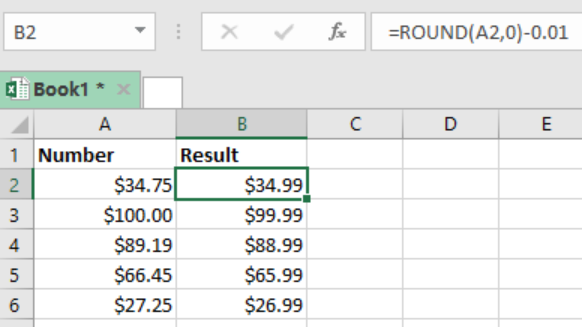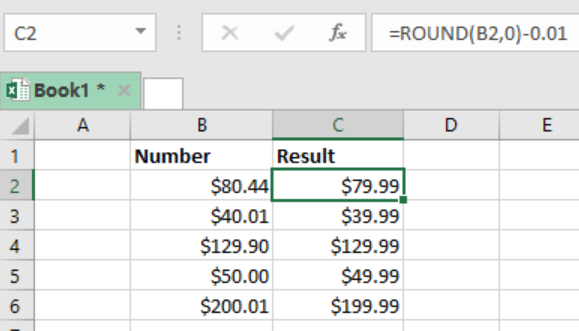Get instant live expert help with Excel or Google Sheets“My Excelchat expert helped me in less than 20 minutes, saving me what would have been 5 hours of work!”

#### Post your problem and you'll get expert help in seconds

Your message must be at least 40 characters
Our professional experts are available now. Your privacy is guaranteed.

# Round a price to end in .99

We can use the Excel ROUND function then subtract to round price to end in .99 value. This post provides a clear guide on how this is done.Figure 1: Using ROUND function to get values that end in .99

## General syntax of the formula

`=ROUND (price, 0) – 0.01`

## Explanation

The excel ROUND function is fundamental when we want to round price values to the nearest .99. in the figure above, the formula in cell B2 is =ROUND (A2,0) -0.01

Given that the value in cell A2 is \$34.75, the value is solved in the following way;

`=ROUND (A2,0) -0.01`

`=ROUND (\$34.75,0) -0.01`

`=\$35-0.01`

`=\$34.99`

Notice that when we use the ROUND function and a zero, the function will round our value to the nearest whole number. Then 0.01 is subtracted from the whole number to get a value ending in .99

## ExampleFigure 2: Example of how ROUND function is used t get values ending in .99

In this example, we want to round the prices in column B to get a result that ends in .99 in column C. to do this, we proceed as follows;

Step 1: Prepare your data, with Number and Result column.

Step 2: In cell C2, enter the formula as `=ROUND (B2,0)-0.01`

Step 3: Press Enter to get the result

Step 4: Copy down the formula to the other cells to get their results

## Instant Connection to an Expert through our Excelchat Service

Most of the time, the problem you will need to solve will be more complex than a simple application of a formula or function. If you want to save hours of research and frustration, try our live Excelchat service! Our Excel Experts are available 24/7 to answer any Excel question you may have. We guarantee a connection within 30 seconds and a customized solution within 20 minutes.

### Did this post not answer your question? Get a solution from connecting with the expert.Another blog reader asked this question today on Excelchat:## Subscribe to Excelchat.coAnother blog reader asked this question today on Excelchat: